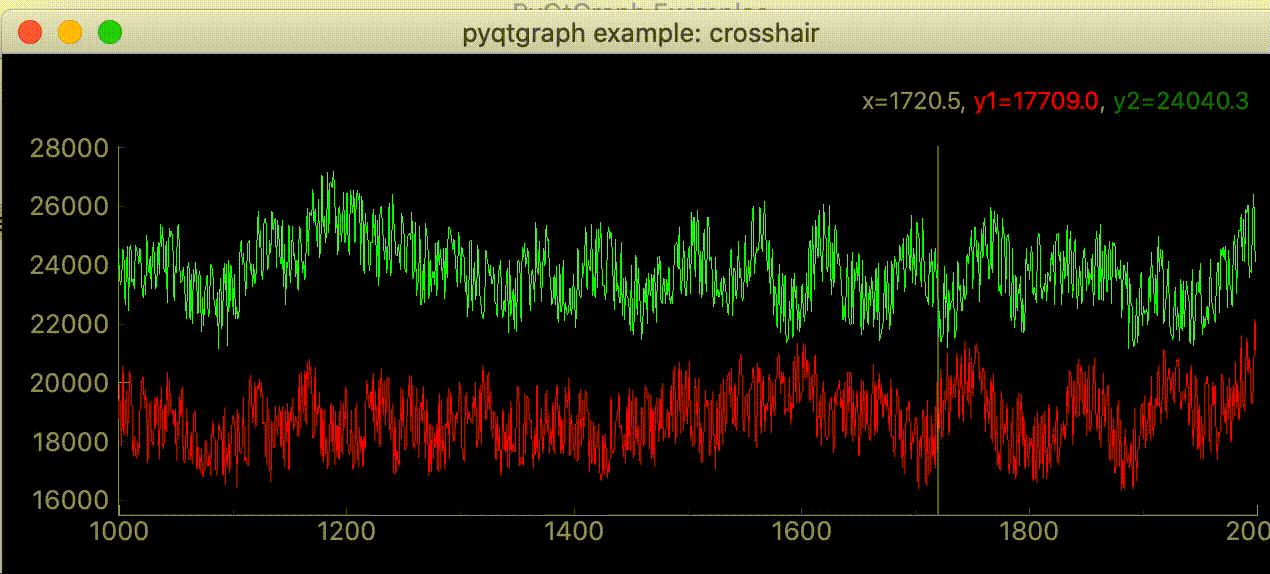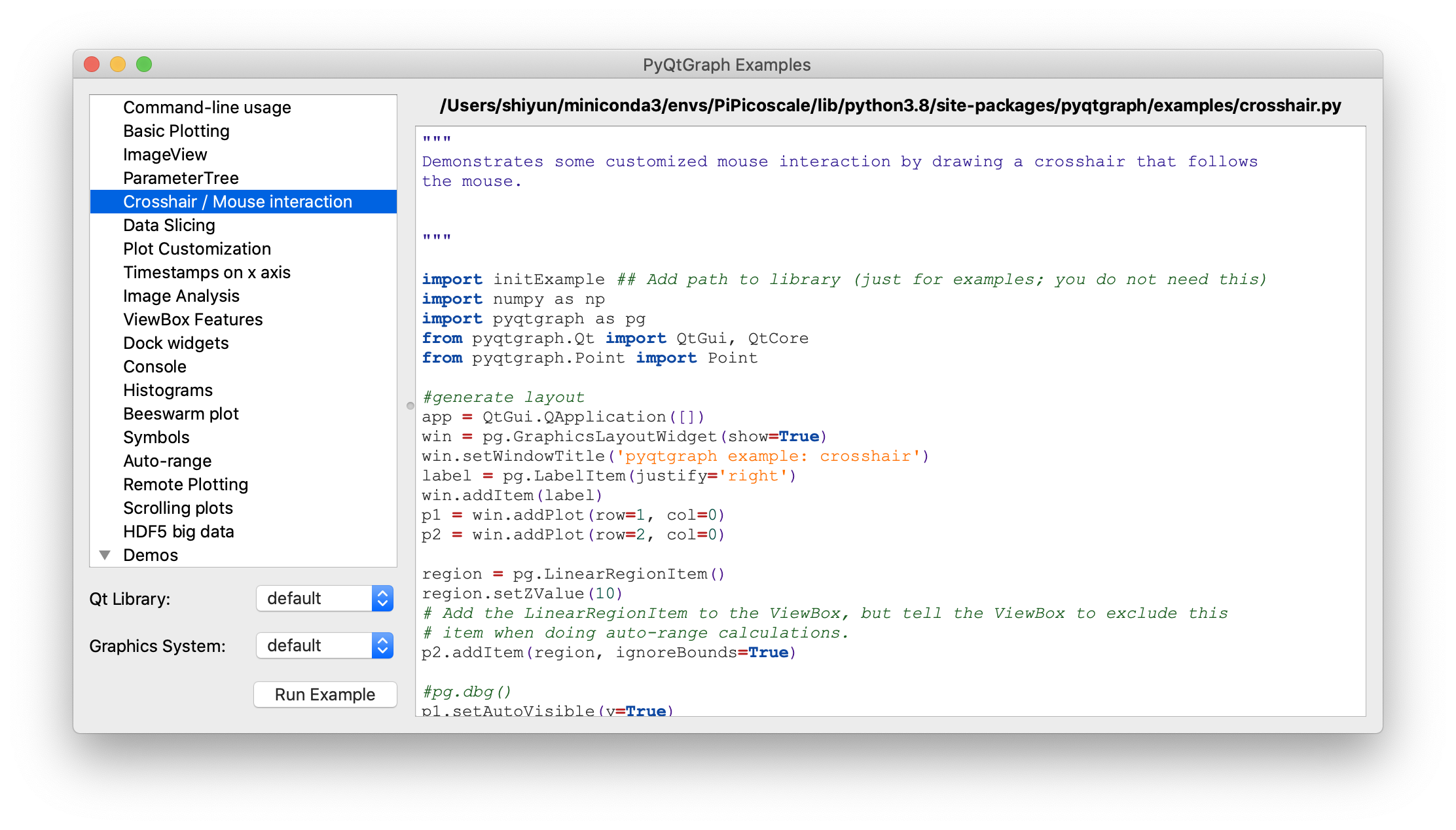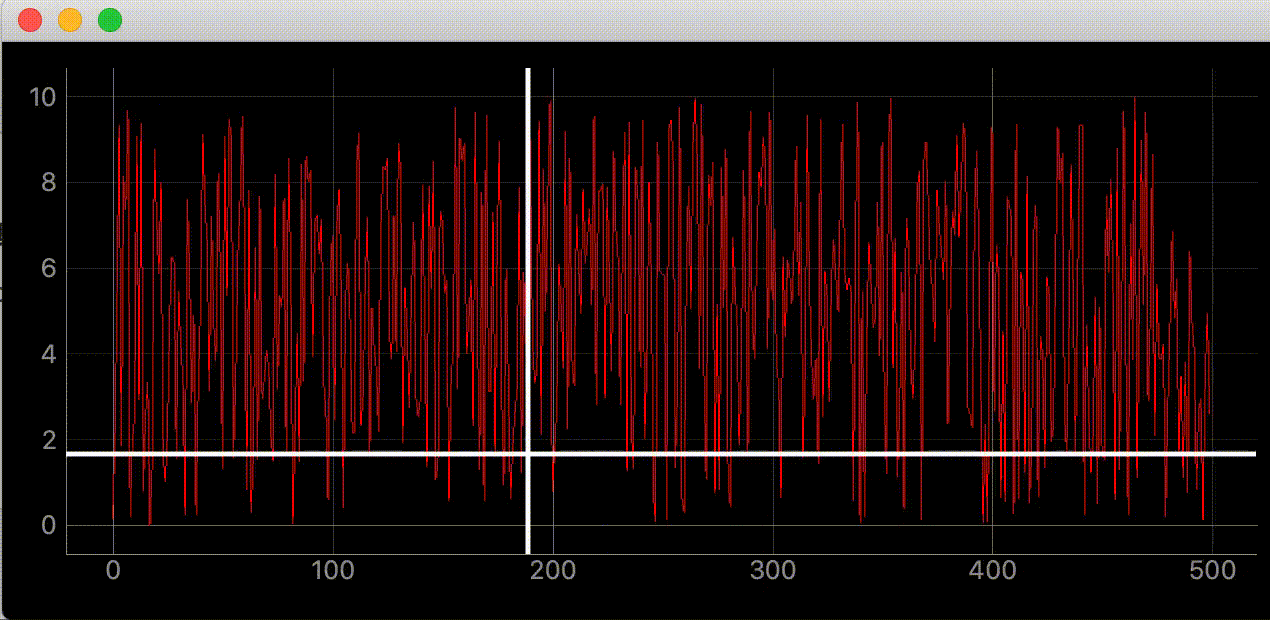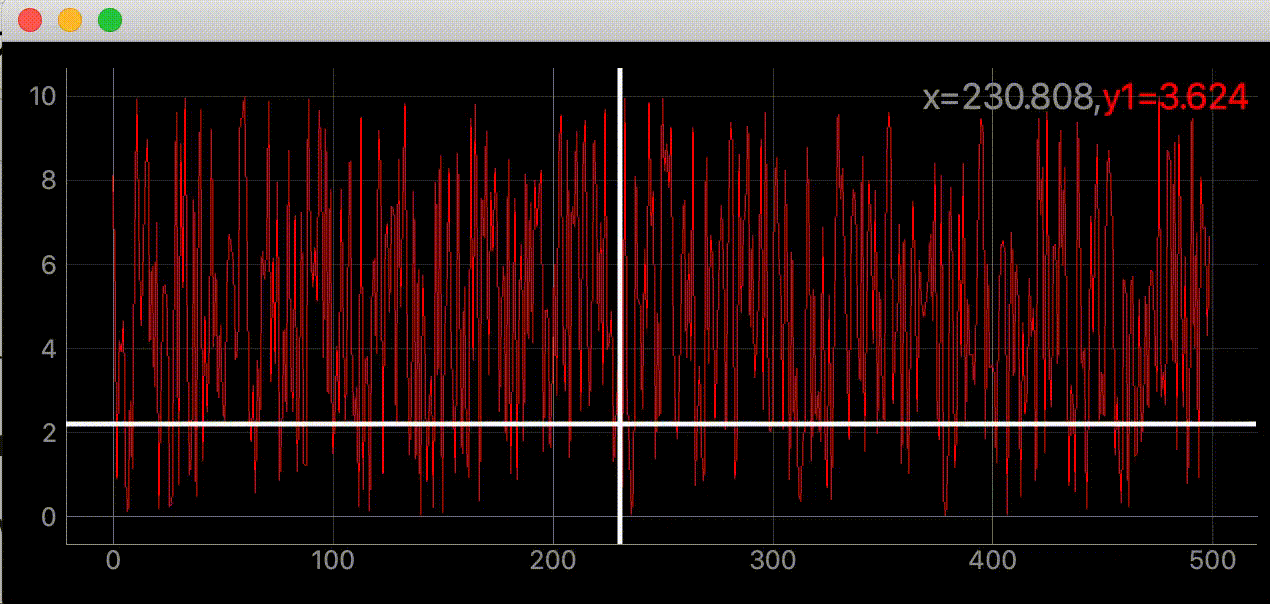# Thing you want to do

I want to create a crosshair that moves according to the cursor in Crosshair / Mouse interaction of `pyqtgraph.exsamples.run ()`.There was a script I saw for the first time in the sample code.

``````proxy = pg.SignalProxy(p1.scene().sigMouseMoved, rateLimit=60, slot=mouseMoved)
``````

SignalProxy creates a crosshair while understanding what it is doing.

# environment

Mac OS Python 3.8.5

PyQt5 5.15.2 PyQt5-sip 12.8.1 pyqtgraph 0.11.1

`pip install PyQt5 PyQt5-sip pyqtgraph`

pyqtgraph.exsamples

``````import pyqtgraph.examples as ex
ex.run()
``````

You can see various sample graphs at. I referred to Crosshair / Mouse interaction this time.SignalProxy Contents of SignalProxy doc

Object which collects rapid-fire signals and condenses them into a single signal or a rate-limited stream of signals. Used, for example, to prevent a SpinBox from generating multiple signals when the mouse wheel is rolled over it.

Emits sigDelayed after input signals have stopped for a certain period of time.

Initialization arguments: signal - a bound Signal or pyqtSignal instance delay - Time (in seconds) to wait for signals to stop before emitting (default 0.3s) slot - Optional function to connect sigDelayed to. rateLimit - (signals/sec) if greater than 0, this allows signals to stream out at a steady rate while they are being received.

An object to use when the same signal is generated in large numbers in a short time. Instead of emitting all the generated signals, only one is emitted at regular intervals.

## argument

--signal: Specify the signal you want to process --delay: The waiting time from receiving the signal to emitting light. The default is 0.3 seconds. When specifying, enter in seconds. --slot: Slot executed when a signal is emitted --rateLimit: Used when you want to set the exact interval at which the signal is emitted. The unit is [Hz]. The default is 0.

## How do you specify the interval?

SignalProxy constructor and the part receiving the signal The slot is executed when `self.timer` times out.

``````    sigDelayed = QtCore.Signal(object)

def __init__(self, signal, delay=0.3, rateLimit=0, slot=None):
"""Initialization arguments:
signal - a bound Signal or pyqtSignal instance
delay - Time (in seconds) to wait for signals to stop before emitting (default 0.3s)
slot - Optional function to connect sigDelayed to.
rateLimit - (signals/sec) if greater than 0, this allows signals to stream out at a
"""

QtCore.QObject.__init__(self)
self.delay = delay
self.rateLimit = rateLimit
self.args = None
self.timer.timeout.connect(self.flush)
self.lastFlushTime = None
self.signal = signal
if slot is not None:
self.blockSignal = False
self.sigDelayed.connect(slot)
self.slot = weakref.ref(slot)
else:
self.blockSignal = True
self.slot = None

"""Received signal. Cancel previous timer and store args to be
forwarded later."""
if self.blockSignal:
return
self.args = args
if self.rateLimit == 0:
self.timer.stop()
self.timer.start(int(self.delay * 1000) + 1)
else:
now = time()
if self.lastFlushTime is None:
leakTime = 0
else:
lastFlush = self.lastFlushTime
leakTime = max(0, (lastFlush + (1.0 / self.rateLimit)) - now)

self.timer.stop()
self.timer.start(int(min(leakTime, self.delay) * 1000) + 1)
``````

### When rateLimit is not specified

The default for rateLimit is 0

``````        if self.rateLimit == 0:
self.timer.stop()
self.timer.start(int(self.delay * 1000) + 1)
``````

The timer is reset to fire `self.delay` seconds after the signal was last received. Since `timer.start ()` is specified in milliseconds, * 1000 is used.

### When rateLimit is specified

``````        else:
now = time()
if self.lastFlushTime is None:
leakTime = 0
else:
lastFlush = self.lastFlushTime
leakTime = max(0, (lastFlush + (1.0 / self.rateLimit)) - now)

self.timer.stop()
self.timer.start(int(min(leakTime, self.delay) * 1000) + 1)
``````

`self.lastFlushTime` is the unix time when the signal was last issued.

#### When enough time has passed since the signal last emitted

Example

• `self.rateLimit` = 5
• `self.lastFlushTime` = 100
• `now` = 102

`leakTime = max(0, (lastFlush + (1.0 / self.rateLimit)) - now)` = max (0, -1.8) and `leakTime` = 0 After that, `self.timer.start (int (min (leakTime, self.delay) * 1000) + 1)`, `self.delay` is larger, so it emits light after delay seconds.

#### When the signal occurs in less than (1 / rateLimit) seconds

Example

• `self.rateLimit` = 5
• `self.lastFlushTime` = 100
• `now` = 100.01

`leakTime = max(0, (lastFlush + (1.0 / self.rateLimit)) - now)` = max (0, 0.19) and `leakTime` = 0.19

Subsequent `self.timer.start (int (min (leakTime, self.delay) * 1000) + 1)` will emit light after `leaktime` seconds if it is larger than` self.delay`.

0.01 seconds have passed since the last issue until now, so 0.01 + 0,19 = 0.2 = 5 [Hz]

## in short

First this is

``````proxy = pg.SignalProxy(p1.scene().sigMouseMoved, rateLimit=60, slot=mouseMoved)
``````

It means to execute `mouseMoved` every` 60 Hz` when the mouse moves (`p1.scene (). SigMouseMoved`).

# code

Now that I know what SignalProxy is doing, I'll use it. Use numpy for plotting. `pip install numpy```````"""Add a crosshair to the graph to follow the mouse cursor"""

import dataclasses
from typing import Optional
import sys

import numpy as np
from PyQt5 import QtWidgets, QtCore
import pyqtgraph as pg

SAMPLE_DATA = np.random.rand(500) * 10

@dataclasses.dataclass
"""Main screen
Attributes #
----------
parent: Optional[QtWidgets.QWidget] default=None
Parent screen
plotter: pyqtgraph.graphicsItems.PlotItem.PlotItem.PlotItem
Main graph
view_box: pyqtgraph.graphicsItems.ViewBox.ViewBox.ViewBox
ViewBox of the main graph
vertical_line: pyqtgraph.graphicsItems.InfiniteLine.InfiniteLine
Vertical line chasing the mouse cursor
horizontal_line: pyqtgraph.graphicsItems.InfiniteLine.InfiniteLine
Horizontal line chasing the mouse cursor
proxy: pyqtgraph.SignalProxy.SignalProxy
Controls the emission of signals that occur when the mouse cursor moves
"""
parent: Optional[QtWidgets.QWidget] = None

def __post_init__(self) -> None:

self.set_proxy()

self.plotter.showGrid(x=True, y=True, alpha=0.8)
self.plotter.plot(SAMPLE_DATA, pen=pg.mkPen('#f00'))

# self.ViewBox of plotter
self.view_box = self.plotter.vb

"""Add a line that moves with the cursor"""
#Since it is hard to see by default, specify the color and width
self.vertical_line = pg.InfiniteLine(angle=90, movable=False, pen=pg.mkPen('#fff', width=5))
self.horizontal_line = pg.InfiniteLine(angle=0, movable=False, pen=pg.mkPen('#fff', width=5))

def set_proxy(self) -> None:
"""Set Signal Proxy"""
self.proxy = pg.SignalProxy(self.plotter.scene().sigMouseMoved, rateLimit=60, slot=self.mouse_moved)

@QtCore.pyqtSlot(tuple)
def mouse_moved(self, evt) -> None:
"""A function that is executed every 60 FPS when the mouse moves
PlotItem.scene().sigMouseMoved returns screen pixel coordinates instead of graph coordinates

Parameters
----------
evt: tuple
Screen pixel coordinates
ex) (PyQt5.QtCore.QPointF(2.0, 44.0),)
"""
#Get screen pixel coordinates
# ex) pos=PyQt5.QtCore.QPointF(2.0, 44.0)
pos = evt
#If pos is the coordinates in the graph
if self.plotter.sceneBoundingRect().contains(pos):
#Get coordinates of graph
# ex) mousePoint=PyQt5.QtCore.QPointF(141.6549821809388, 4.725564511858496)
mouse_point = self.view_box.mapSceneToView(pos)
#Mouse the line_Move to the coordinates of point
# ex) mouse_point.x()=46.13389087421787
self.vertical_line.setPos(mouse_point.x())
# ex) mouse_point.y()=9.535145662930628
self.horizontal_line.setPos(mouse_point.y())

def main() -> None:
app = QtWidgets.QApplication(sys.argv)
window.show()
sys.exit(app.exec_())

if __name__ == "__main__":
main()

``````

## Display coordinates in the upper right``````"""Add a crosshair to the graph to follow the mouse cursor"""

import dataclasses
from typing import Optional
import sys

import numpy as np
from PyQt5 import QtWidgets, QtCore
import pyqtgraph as pg

SAMPLE_DATA = np.random.rand(500) * 10

@dataclasses.dataclass
"""Main screen
Attributes #
----------
parent: Optional[QtWidgets.QWidget] default=None
Parent screen
plotter: pyqtgraph.graphicsItems.PlotItem.PlotItem.PlotItem
Main graph
view_box: pyqtgraph.graphicsItems.ViewBox.ViewBox.ViewBox
ViewBox of the main graph
vertical_line: pyqtgraph.graphicsItems.InfiniteLine.InfiniteLine
Vertical line chasing the mouse cursor
horizontal_line: pyqtgraph.graphicsItems.InfiniteLine.InfiniteLine
Horizontal line chasing the mouse cursor
proxy: pyqtgraph.SignalProxy.SignalProxy
Controls the emission of signals that occur when the mouse cursor moves
"""
parent: Optional[QtWidgets.QWidget] = None

def __post_init__(self) -> None:

self.set_proxy()

self.plotter.showGrid(x=True, y=True, alpha=0.8)
self.plotter.plot(SAMPLE_DATA, pen=pg.mkPen('#f00'))

# self.ViewBox of plotter
self.view_box = self.plotter.vb

self.label = pg.LabelItem(justify='right')

"""Add a line that moves with the cursor"""
#Since it is hard to see by default, specify the color and width
self.vertical_line = pg.InfiniteLine(angle=90, movable=False, pen=pg.mkPen('#fff', width=5))
self.horizontal_line = pg.InfiniteLine(angle=0, movable=False, pen=pg.mkPen('#fff', width=5))

def set_proxy(self) -> None:
"""Set Signal Proxy"""
self.proxy = pg.SignalProxy(self.plotter.scene().sigMouseMoved, rateLimit=60, slot=self.mouse_moved)

@QtCore.pyqtSlot(tuple)
def mouse_moved(self, evt) -> None:
"""A function that is executed every 60 FPS when the mouse moves
PlotItem.scene().sigMouseMoved returns screen pixel coordinates instead of graph coordinates

Parameters
----------
evt: tuple
Screen pixel coordinates
ex) (PyQt5.QtCore.QPointF(2.0, 44.0),)
"""
#Get screen pixel coordinates
# ex) pos=PyQt5.QtCore.QPointF(2.0, 44.0)
pos = evt
#If pos is the coordinates in the graph
if self.plotter.sceneBoundingRect().contains(pos):
#Get coordinates of graph
# ex) mousePoint=PyQt5.QtCore.QPointF(141.6549821809388, 4.725564511858496)
mouse_point = self.view_box.mapSceneToView(pos)

# SAMPLE_If the coordinates are in DATA, x,Display y value
index = int(mouse_point.x())
if 0 < index < len(SAMPLE_DATA):
self.label.setText(
f"<span style='font-size: 18pt'>x={mouse_point.x():.3f},"
f"<span style='color: red'>y1={SAMPLE_DATA[index]:.3f}</span>")

#Mouse the line_Move to the coordinates of point
# ex) mouse_point.x()=46.13389087421787
self.vertical_line.setPos(mouse_point.x())
# ex) mouse_point.y()=9.535145662930628
self.horizontal_line.setPos(mouse_point.y())

def main() -> None:
app = QtWidgets.QApplication(sys.argv)